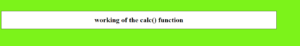Related Articles
How to Set Calc Viewport Units Workaround in CSS ?
• Last Updated : 30 Jun, 2020

In the HTML webpage, when applying values to the CSS properties, the calculations are performed by using calc() function.

Syntax:

```element {

// CSS property
property : calc( expression)
}
```

Properties: The calc() function takes parameters in the form of a single expression. The value becomes the result of the expression. Even the expression be the combination of many operators follow the standard precedence rules.

• – Subtraction
• * Multiplication. Any of the arguments should be a number
• / Division. The right-hand side should be a number

One must be careful while writing the syntax and must take care of the following points.

1. The + and operator must be surrounded by white space i.e. height(100%-30px) is considered to be invalid but height(100% – 30px) is a valid expression. For the / and * operator, the white spacing is not necessary but is highly recommended.
2. Division by 0 results in an error.

Example 1:

 ` ` `<``html` `lang``=``"en"``> ` ` `  `<``head``> ` `    ``<``meta` `charset``=``"UTF-8"``> ` `    ``<``meta` `name``=``"viewport"` `content``= ` `        ``"width=device-width, initial-scale=1.0"``> ` `    ``<``title``> ` `        ``CSS Calc Viewport Units Workaround ` `    `` ` `     `  `    ``<``style``> ` `        ``body { ` `            ``background-color: rgb(30, 240, 30); ` `        ``} ` ` `  `        ``h1 { ` `            ``color: rgb(30, 240, 30); ` `        ``} ` ` `  `        ``div { ` `            ``width: calc(100% - 100px); ` ` `  `            ``/* Using the calc() func to change the  ` `               ``width of the div element by 100px */ ` `            ``border: 1px solid black; ` `            ``background-color: white; ` `            ``margin-top: 50px; ` `            ``text-align: center; ` `        ``} ` `    `` ` ` ` ` `  `<``body``> ` `    ``<``div``> ` `        ``<``h1``>working of the calc() function ` `    `` ` ` ` ` `  ` `

output:Example 2:

 ` ` `<``html` `lang``=``"en"``> ` ` `  `<``head``> ` `    ``<``meta` `charset``=``"UTF-8"``> ` `    ``<``meta` `name``=``"viewport"` `content``= ` `        ``"width=device-width, initial-scale=1.0"``> ` ` `  `    ``<``title``> ` `        ``CSS Calc Viewport Units Workaround ` `    `` ` ` `  `    ``<``style``> ` `        ``body { ` `            ``background-color: rgb(30, 240, 30); ` `        ``} ` ` `  `        ``h1 { ` `            ``color: rgb(30, 240, 30); ` ` `  `            ``/* Using the calc() func to change  ` `            ``the font size of the h1 element */ ` `            ``font-size: calc(1.5rem + 3vw); ` `        ``} ` ` `  `        ``div { ` `            ``width: calc(100% - 100px); ` `            ``border: 1px solid black; ` `            ``background-color: white; ` `            ``margin-top: 50px; ` `            ``text-align: center; ` `        ``} ` `    `` ` ` ` ` `  `<``body``> ` `    ``<``div``> ` `        ``<``h1``> working of the calc() function ` `    `` ` ` ` ` `  ` `

Output:My Personal Notes arrow_drop_up
Recommended Articles
Page :